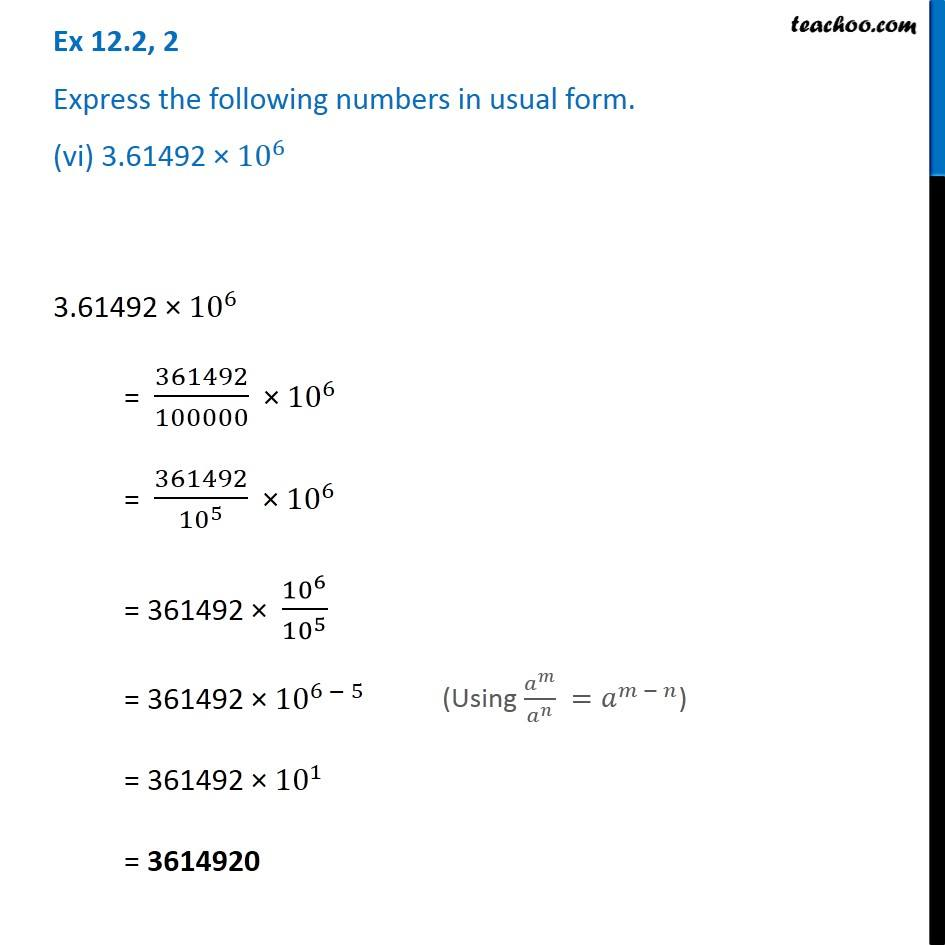Ex 12.2

Chapter 12 Class 8 Exponents and Powers
Serial order wiseGet live Maths 1-on-1 Classs - Class 6 to 12

### Transcript

Ex 12.2, 2 Express the following numbers in usual form. (vi) 3.61492 × 〖10〗^6 3.61492 × 〖10〗^6 = 361492/100000 × 〖10〗^6 = 361492/〖10〗^5 × 〖10〗^6 = 361492 × 〖10〗^6/〖10〗^5 = 361492 × 〖10〗^(6 − 5) = 361492 × 〖10〗^1 = 3614920 (Using 𝑎^𝑚/𝑎^𝑛 =𝑎^(𝑚 − 𝑛))